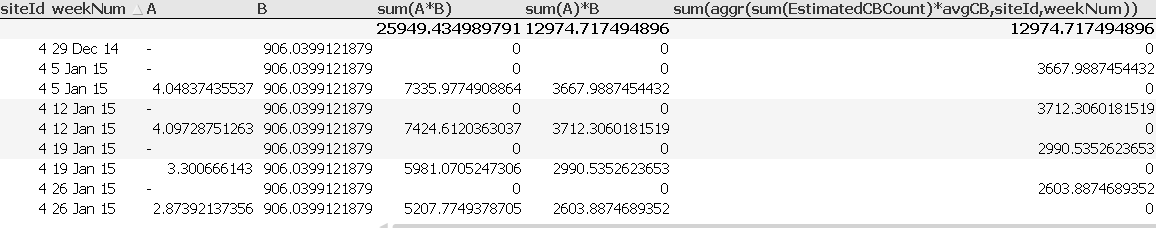# New to QlikView

Discussion board where members can get started with QlikView.

Announcements
Leverage your QlikView investment to modernize BI – see how! Join Group
cancel
Showing results for
Did you mean:
HighlightedContributor III

## Strange behavior with sum() and aggr()

Hi all,

I'm working on this straight table:I've 2 fields, A and B. B has the same value for all rows, but A differs and sometimes is null. I wish to calculate sum of A*B for every siteId and weekNum.

I tried doing this with 3 different expressions:

1. sum(A)*B : gave the correct results.
2. sum(A*B) : gave a result twice as large as that of sum(A)*B
3. sum(aggr(sum(A*B,siteid))) : gives the correct numbers, but on the wrong lines...

Any idea why only sum(A)*B gives the correct results?

Thank  you.

Tags (2)
4 Replies
HighlightedMVP & Luminary

## Re: Strange behavior with sum() and aggr()

I think your expression should be: sum(A) * only(B). Your second expression aggregated B and led therefore to the wrong results. The third expression had a incorrect syntax and should be rather like: sum(aggr(sum(A)*only(B),siteid)).

But why will you use them? Also why didn't you hide NULL in field A?

To aggeregations and especially with the aggr-function see here:

It’s all Aggregations

AGGR...

When should the Aggr() function NOT be used?

- Marcus

HighlightedContributor III

## Re: Strange behavior with sum() and aggr()

I figured that in this specific case

sum(A)*B = sum(A*B), since B is identical for all siteID's.

It's basically just like

1*2+2*2+3*2 = (1+2+3)*2

Does QlikView's sum() work in a different way?

HighlightedMVP & Luminary

## Re: Strange behavior with sum() and aggr()

This isn't the point:

F1     F2     F3     sum(F1*F2)

1        -        2          -

1        2       2          2 * (2 + 2) = 8 // and not on row-level 2 * 2 = 4

....

This results because F1 is for both rows identical.

- Marcus

HighlightedContributor

## Re: Strange behavior with sum() and aggr()

Hey..am trying to replicate and understand the abnormal behaviour. But I am unabel to replicate.

Below is waht I used. Am I missing something.

Set NullInterpret = 'A'

A, B, C

1,2,3

2,A,3

3,3,3

4,A,3

5,4,3

]

;

and my output for sum(B*C) is coming correct.

I am getting curious to replicate whats happenign above,